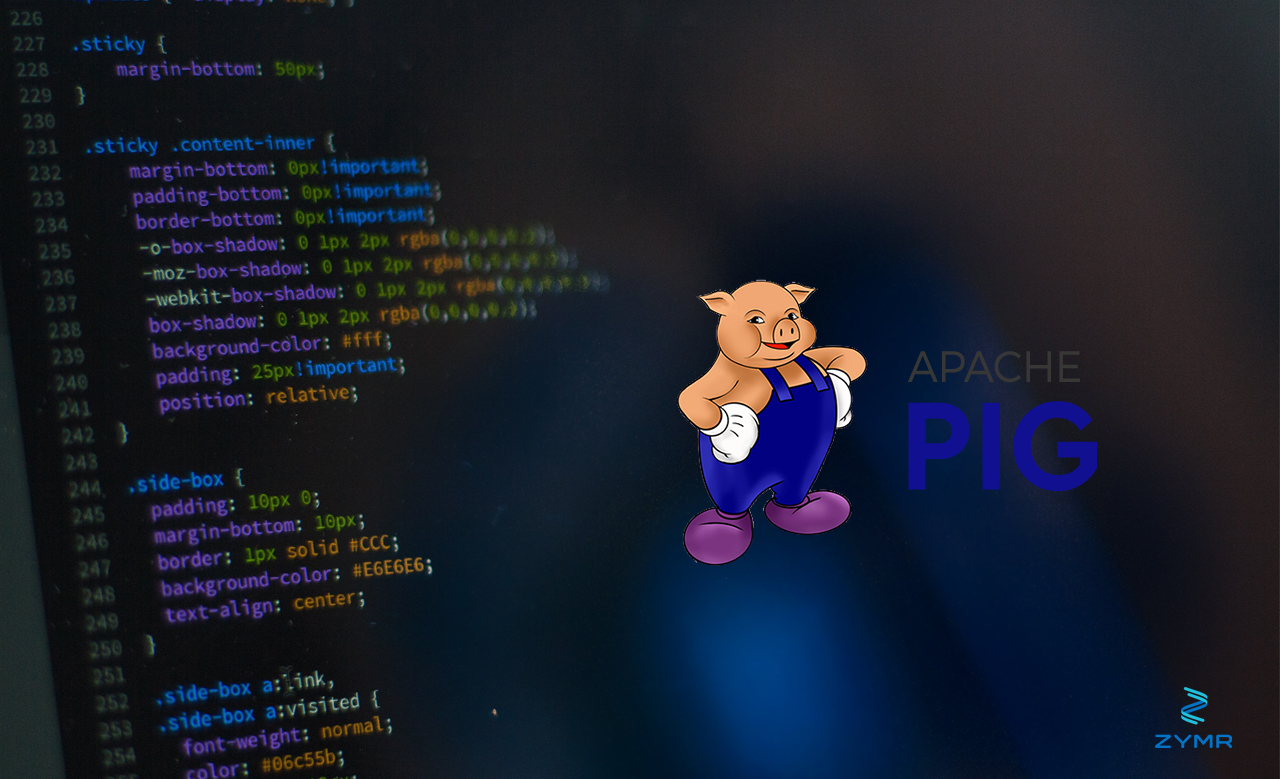Pig does not have the concept of an intersection.  Instead the usual approach to seeing whether there is common data in two sets A and B is to join them and then see which element in A does not exist in B.  That looks kind of messy and one wonders why that is not part of the language.

Here we look at some of those operations in Pig.

First we load comma delimited data.  We are using two very small data sets so that the reader can easily visualize the concepts.

```a = load '/root/Downloads/a.csv' USING PigStorage(',') as (number,text);
(1,one)
(2,two)
(3,three)

(1,ONE)
(2,TWO)
(3,THREE)```

The JOIN Operation

First we do a join. A join by itself is called an inner join. You can think of this as similar to what mathematicians would call this the dot product, which is where you multiply every element in one vector by every vector in another.  But here we do not do any multiplication.  We just stick to sets side-by-side.  And it only joins elements when they have a common element that we select.  That element in this case we take to be number.

x = JOIN A by number, B by number;

This did exactly what is sounded like it would do, which was to take each tuple element and create a new tuple element by simply sticking the two together.

```(1,one,1,ONE)
(2,two,2,TWO)
(3,three,3,THREE)```

Not let’s make the sets unequal sizes by adding 4 to the left.  Here is our new set A and the original B:

 A B (1,one) (2,two) (3,three) (4,four) (1,ONE) (2,TWO) (3,THREE)

Now if we join A and B we have:

```(1,one,1,TWO)
(1,one,1,ONE)
(3,three,3,THREE)```

It dropped the 4 because there was no corresponding 4 element in the B set.  If we wanted to add it then we would use a left outer join. That means on the left hand side include every element including those with no corresponding entry on the right.  Of course there is a right outer join as well.

x = JOIN A by number left outer, B by number;

dump x

Notice that we added the words left outer.  Now we have:

```(1,one,1,ONE)
(2,two,2,TWO)
(3,three,3,THREE)
(4,four,)```

Now let’s put 3 in the left 2 times:

```(1,one)
(2,two)
(3,three)
(3,III)
(4,four)```

In the inner join it matches up every row that has a common element.  So it matched up the 3 twice since it occurs twice on the left and once on the right.

```(1,one,1,ONE)
(2,two,2,TWO)
(3,III,3,THREE)
(3,three,3,THREE)```

The Group Operation

Here we introduce the concept of a bag, which is a group of tuples. Here we are not joining anything.  In the next section, we use cogroup, which both groups and joins.  Here we want to take one set of data, in this case A, and then group tuples that have some common element into a new tuple.  The resulting data structure is (common element, bag of tuples).

```g = GROUP a by number;

(1,{(1,one)})
(2,{(2,two)})
(3,{(3,III),(3,three)})
(4,{(4,four)})```

The CoGroup Operation

CoGroup is the same concept as join except it does not create a new tuple.  Instead it creates a tuple of 3 elements:  the element joined by, the left tuple, and the right tuple.

```x =cogroup a by number, b by number;

(1,{(1,one)},{(1,ONE)})

(2,{(2,two)},{(2,TWO)})

(3,{(3,III),(3,three)},{(3,THREE)})

(4,{(4,four)},{})```

If all of this seems abstract, just know that it is used to build sets based on some common element.  Like “all customers who buy more than x dollars per month.”  So in that case it becomes useful and applicable to business.

This article was authored by Mayur Sonukale, who is a Principal Engineer at Zymr.